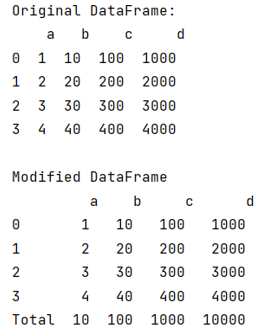# Appending Column Totals to a Pandas DataFrame

Given a DataFrame with numerical values, we need to append a row that represents the sum of each column. By Pranit Sharma Last updated : September 25, 2023

Pandas is a special tool that allows us to perform complex manipulations of data effectively and efficiently. Inside pandas, we mostly deal with a dataset in the form of DataFrame. DataFrames are 2-dimensional data structures in pandas. DataFrames consist of rows, columns, and data.

## Appending Column Totals to a Pandas DataFrame

To append a row consisting of the total of column values, we will first create a new column and assign its values as the use of the sum() method. It returns the sum of the values over the requested axis.

The syntax of the sum() method is:

```DataFrame.sum(axis=0, skipna=True, numeric_only=False, min_count=0, **kwargs)
```
Note

To work with pandas, we need to import pandas package first, below is the syntax:

```import pandas as pd
```

Let us understand with the help of an example,

## Python program to append column totals to a pandas dataframe

```# Importing pandas package
import pandas as pd

# Creating a Dictionary
d = {
'a':[1,2,3,4],
'b': [10,20,30,40],
'c':[100,200,300,400],
'd': [1000,2000,3000,4000]
}

# Creating a DataFrame
df = pd.DataFrame(d)

# Display original DataFrame
print("Original DataFrame:\n",df,"\n")

# Creating new column and assigning sum of column values
df.loc['Total']= df.sum(numeric_only=True, axis=0)

# Display result
print("Modified DataFrame\n",df)
```

### Output

The output of the above program is: